# RS Aggarwal Solutions for Class 8 Maths Chapter 23 - Line Graphs and Linear Graphs

RS Aggarwal Solutions for Class 8 Maths Chapter 23- Line Graphs and Linear Graphs, are provided here. Our expert faculty team has prepared solutions in order to help you with your exam preparation to acquire good marks in Maths. If you wish to secure an excellent score, solving RS Aggarwal Class 8 Solutions is an utmost necessity. These solutions will help you in gaining knowledge and strong command over the subject. Practising the textbook questions will help you in analyzing your level of preparation and knowledge of the concept.

In order to help you understand and solve the problems, we at BYJU’S have designed these solutions in a very lucid and clear manner that helps students solve problems in the most efficient possible ways. Here RS Aggarwal Solutions for Class 8 Chapter 23 contains answers which are solved in detail. Download pdf of Class 8 Chapter 23 in their respective links.

## Download PDF of RS Aggarwal Solutions for Class 8 Maths Chapter 23 – Line Graphs and Linear Graphs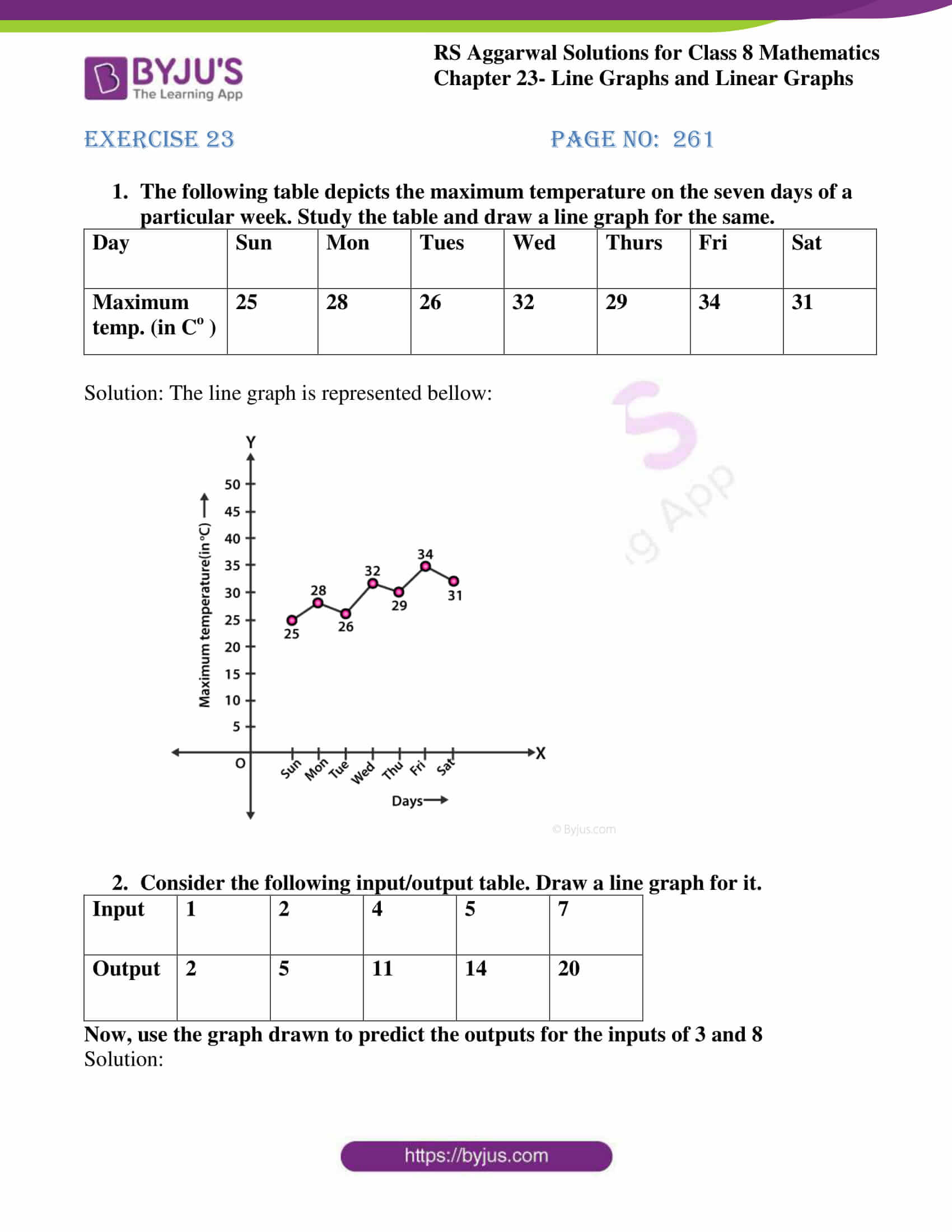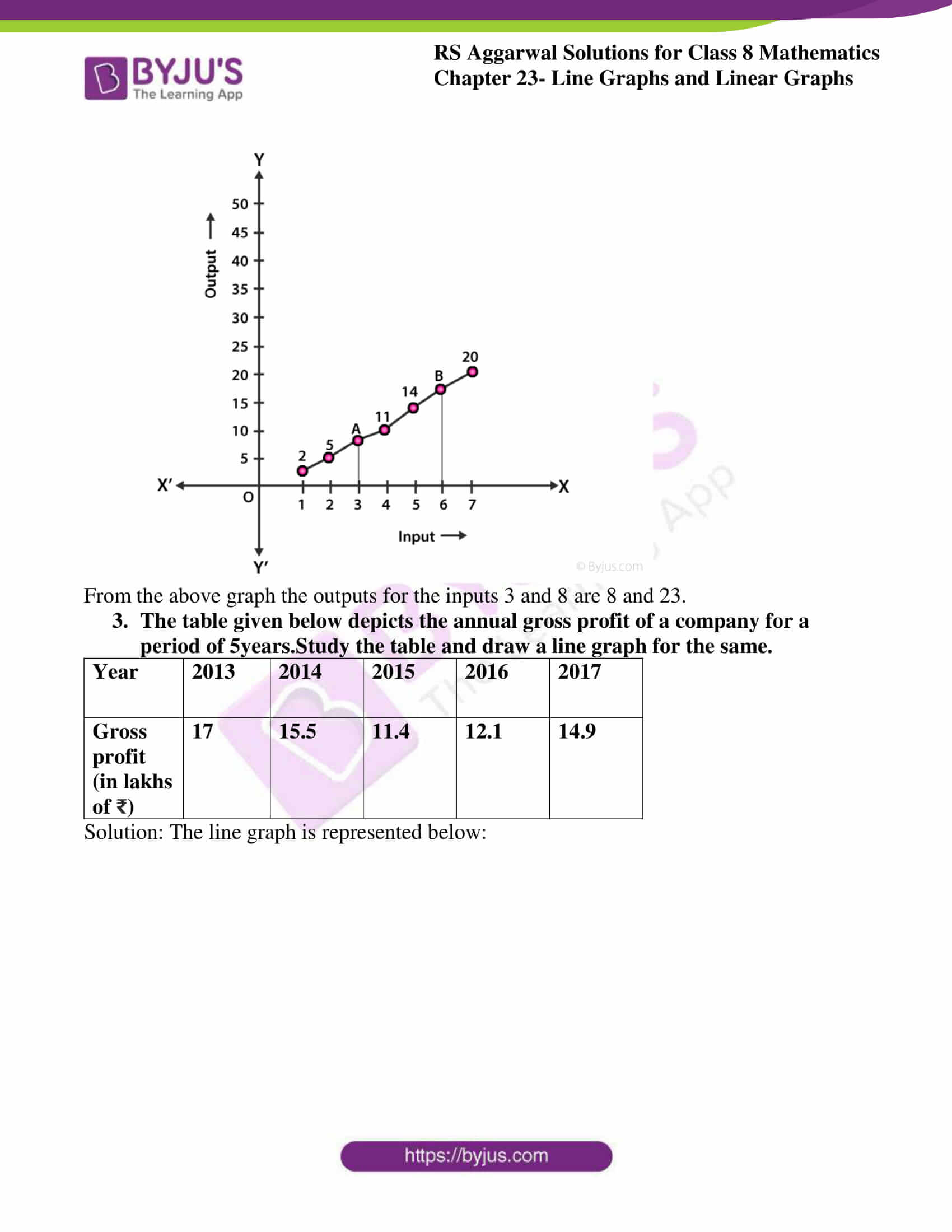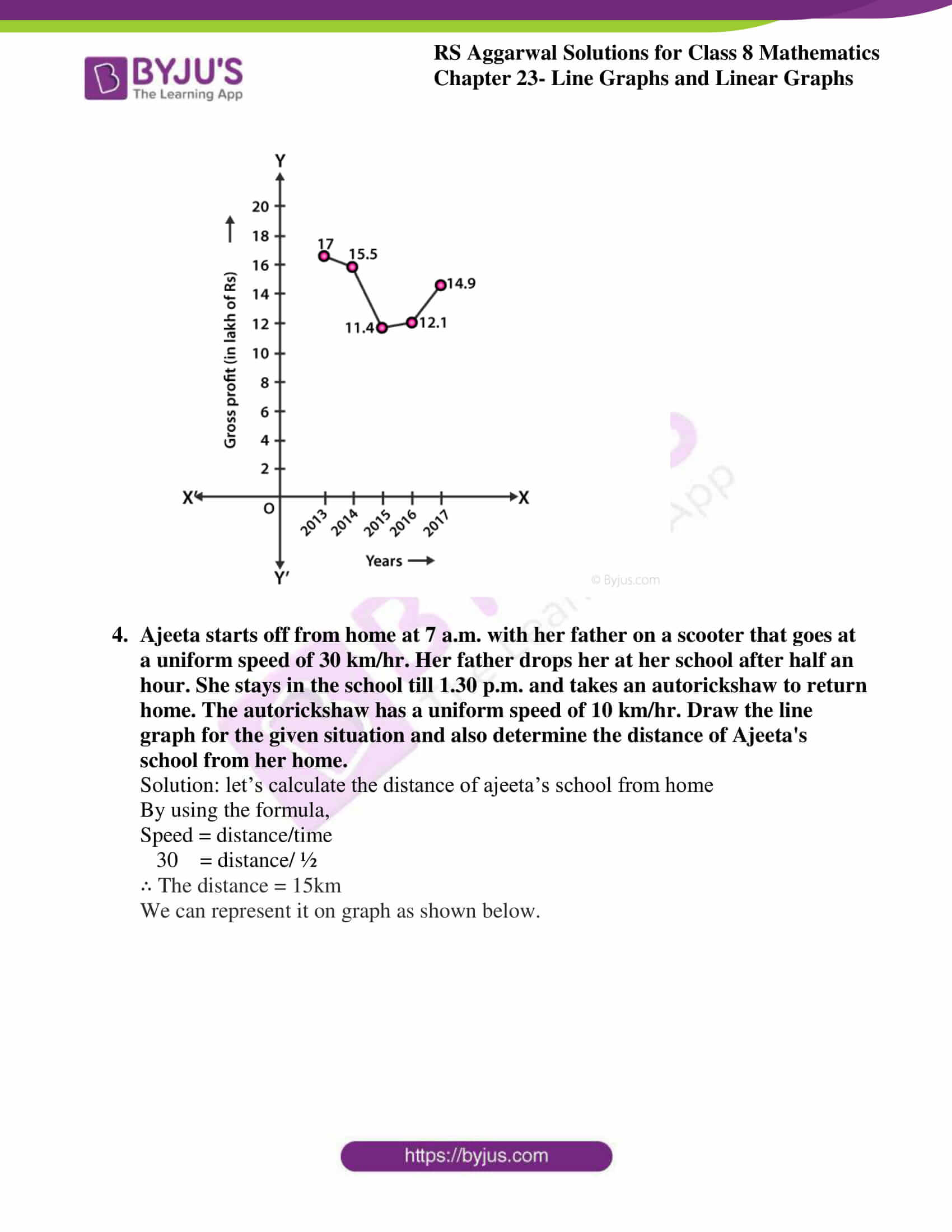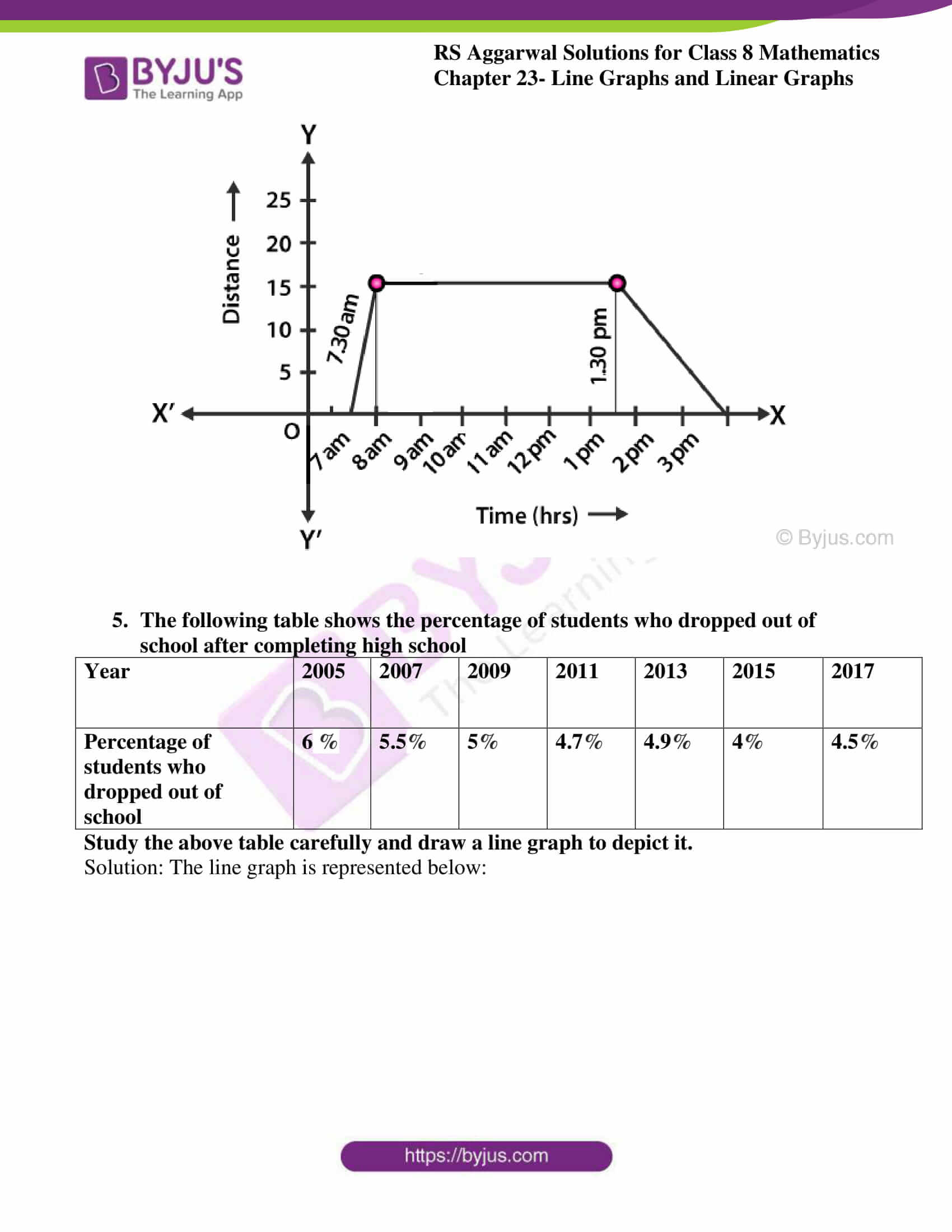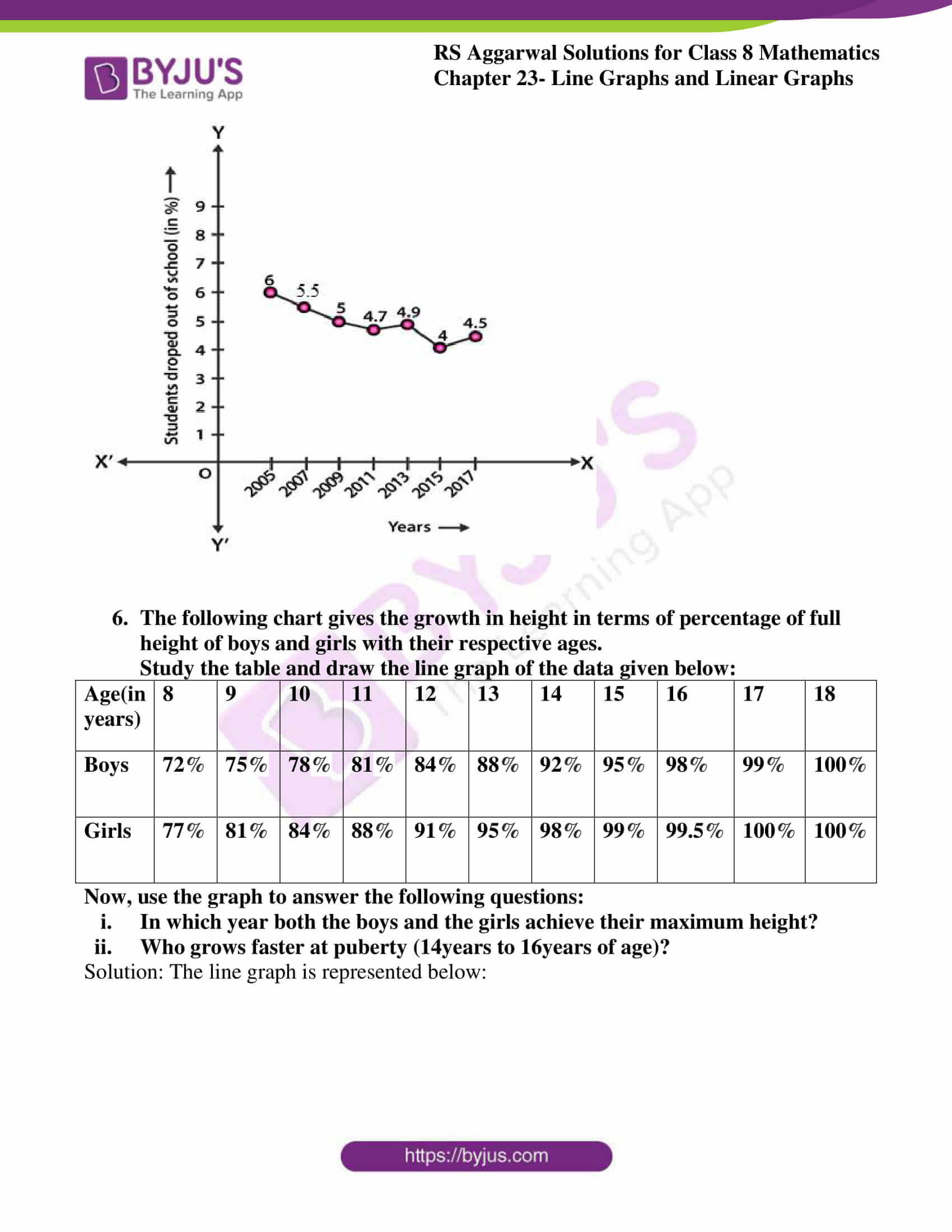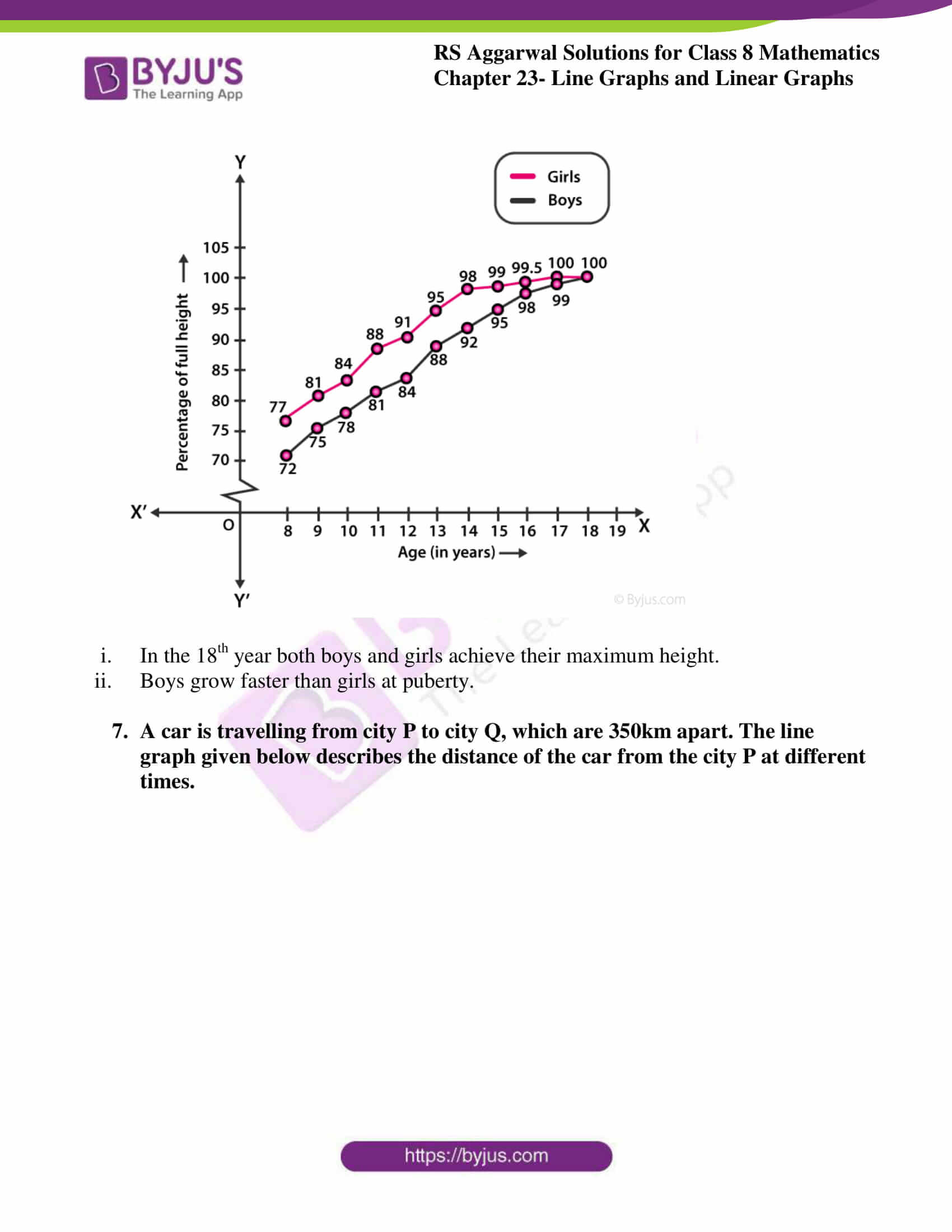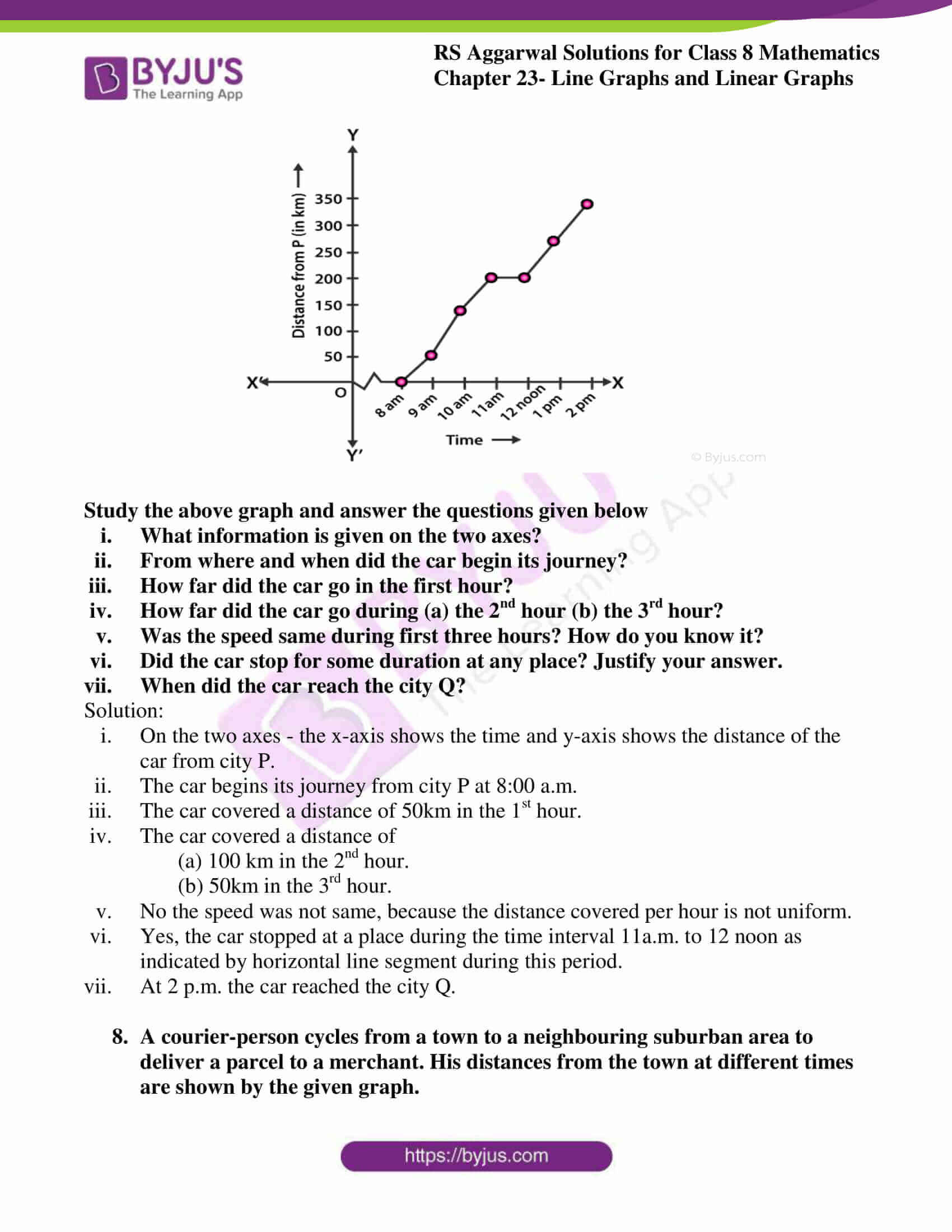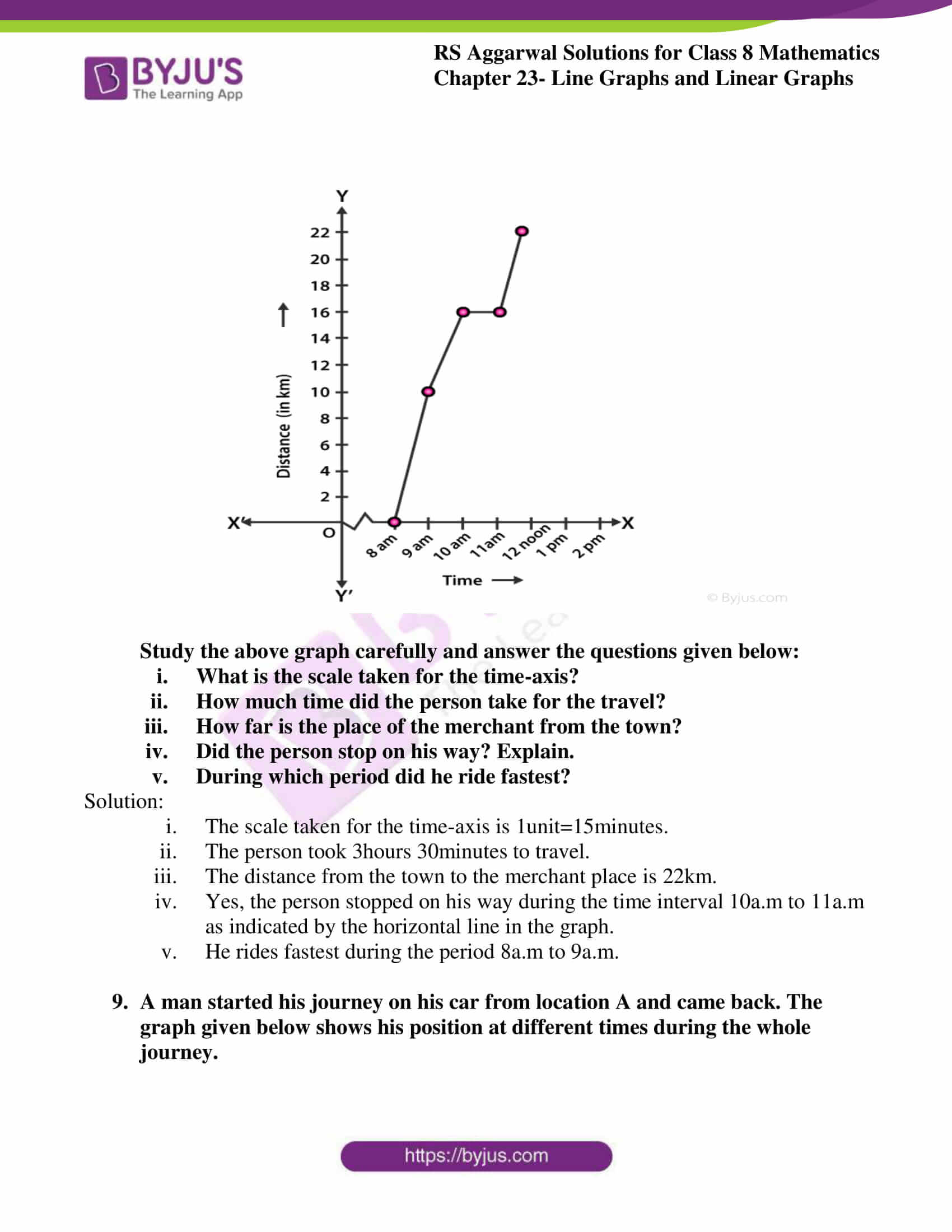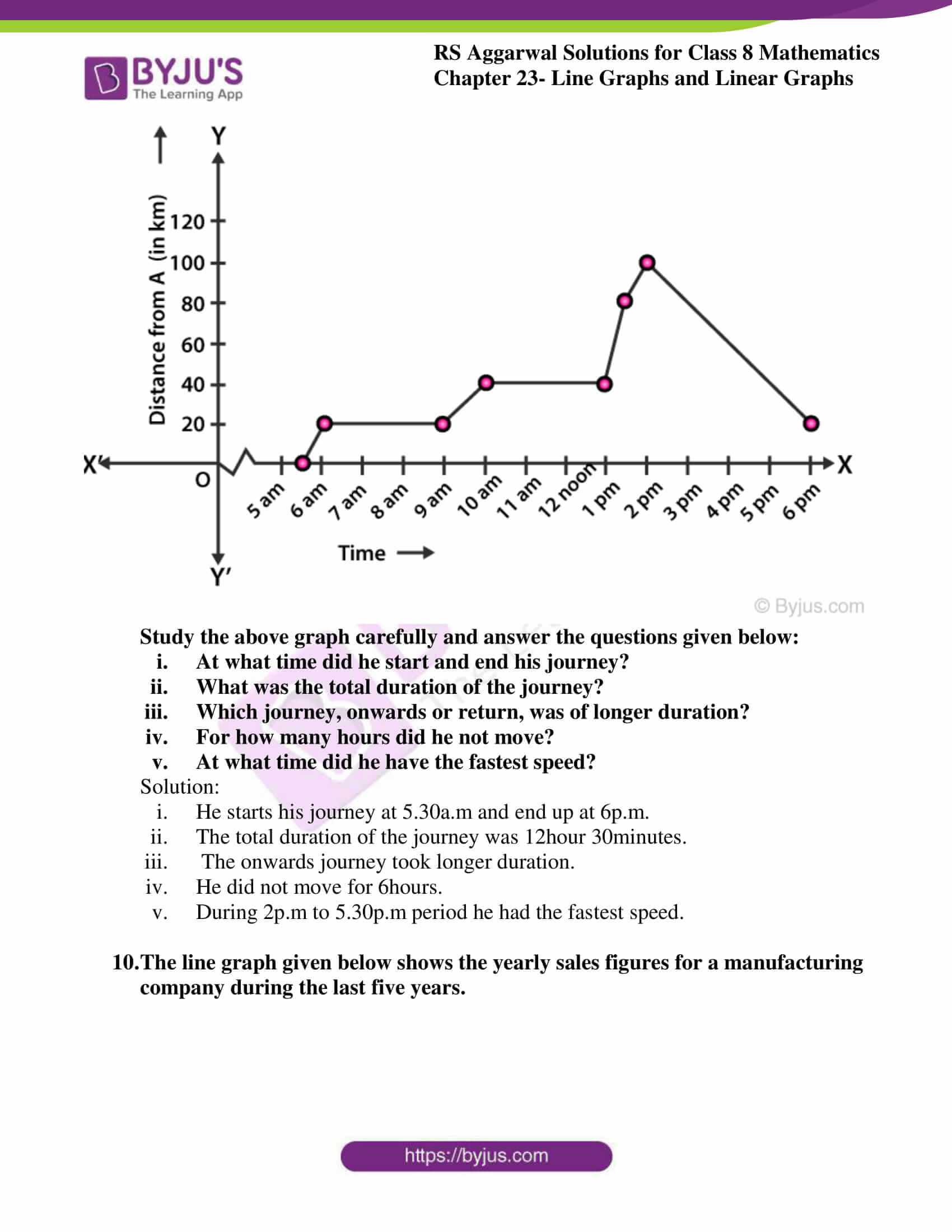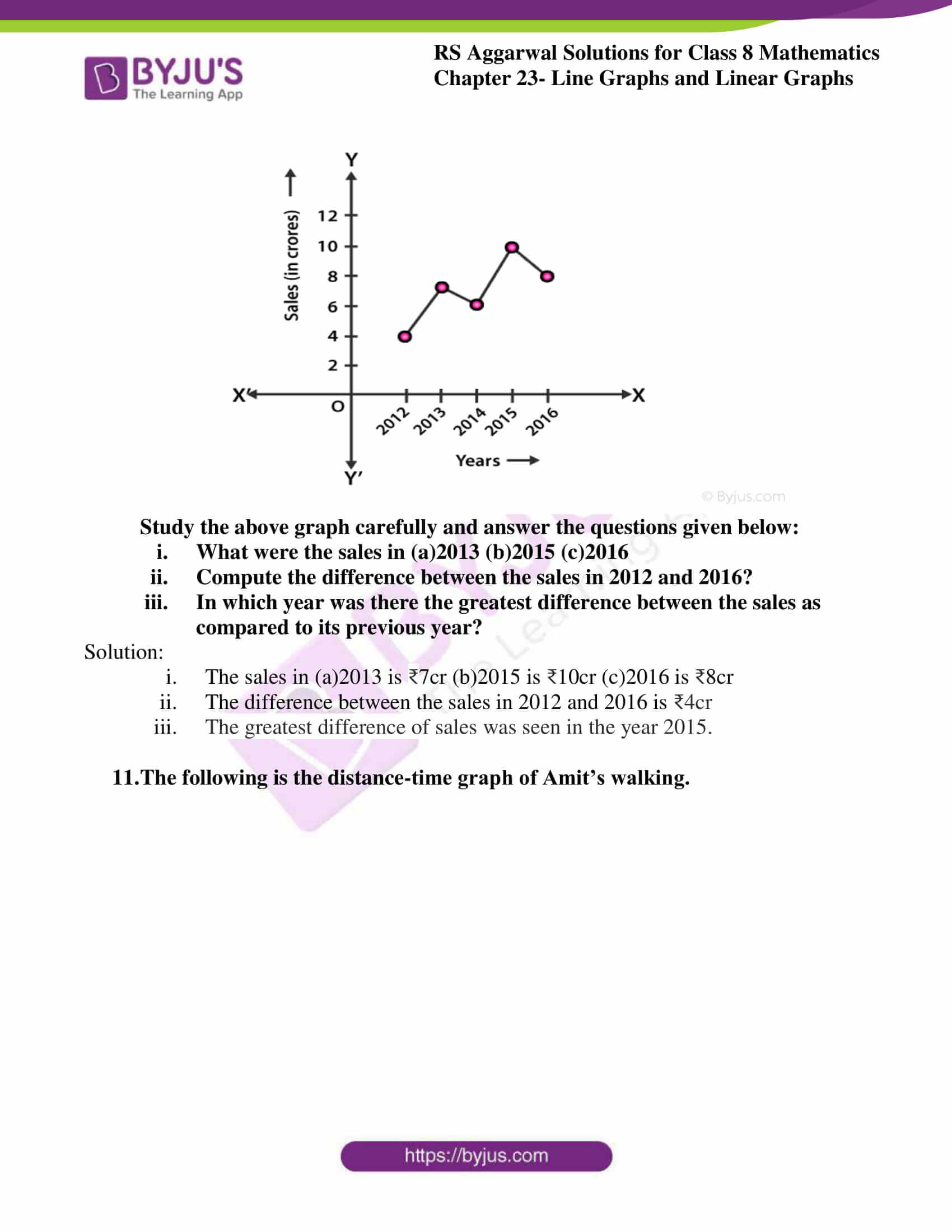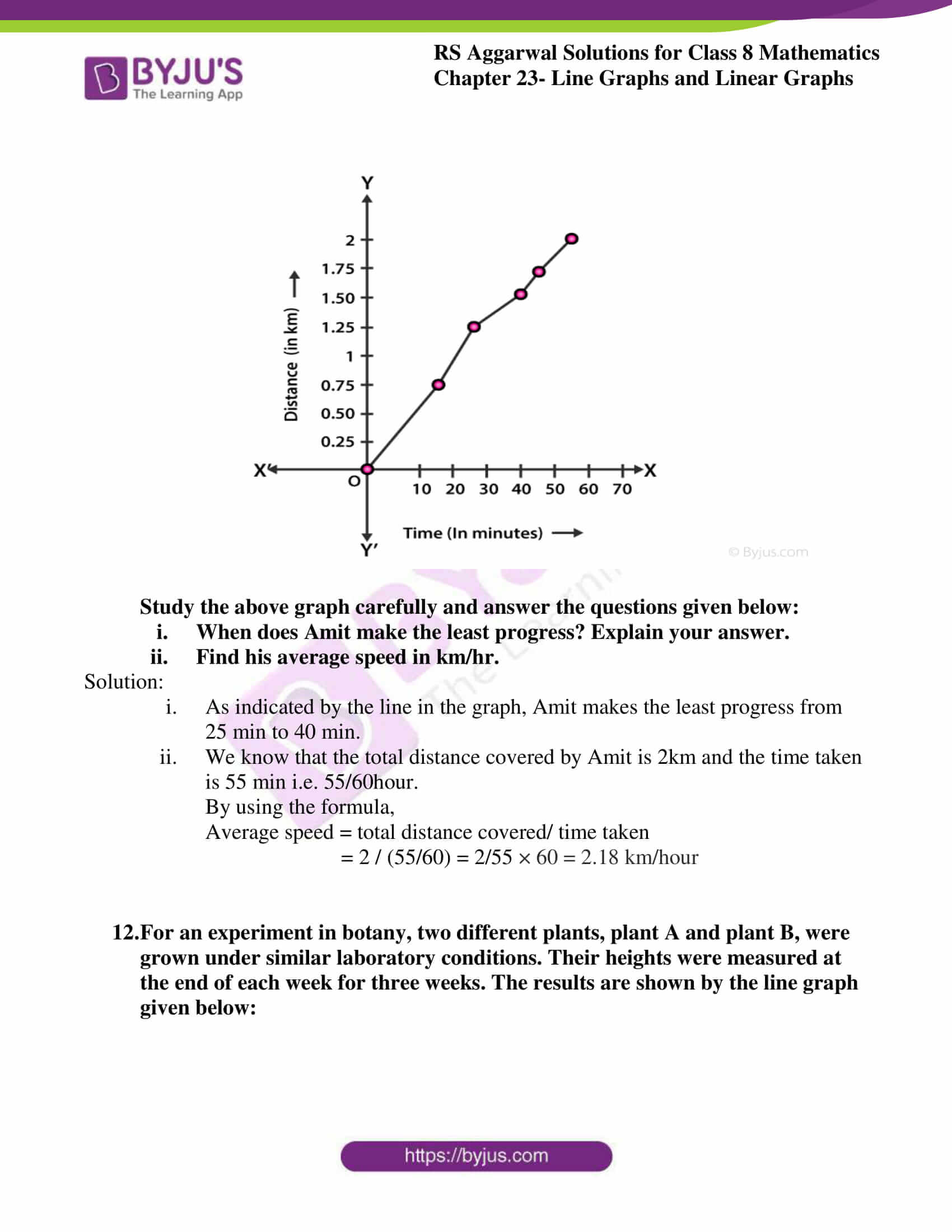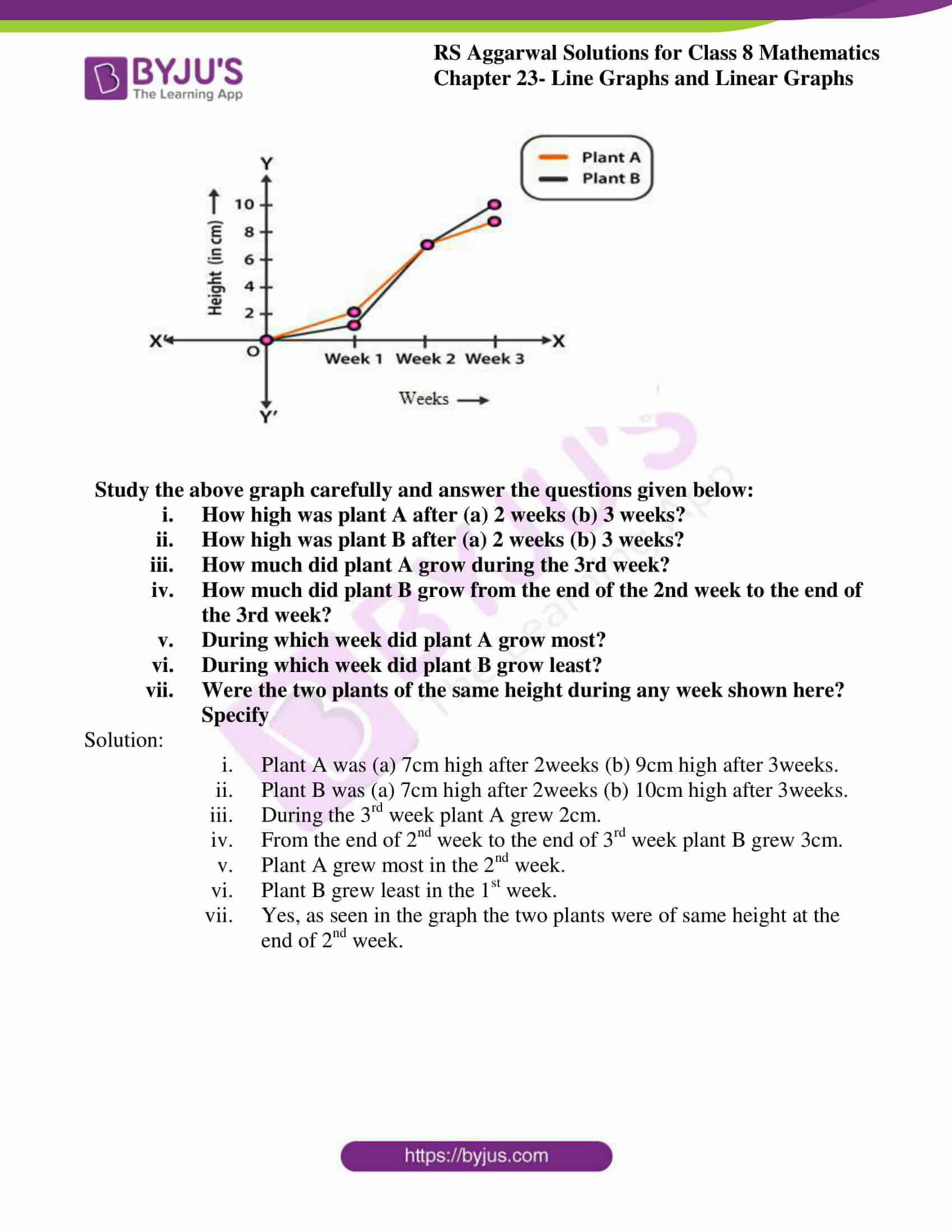## Access answers to Maths RS Aggarwal Solutions for Class 8 Chapter 23 – Line Graphs and Linear Graphs

Exercise 23 Page No: 261

1. The following table depicts the maximum temperature on the seven days of a particular week. Study the table and draw a line graph for the same.

 Day Sun Mon Tues Wed Thurs Fri Sat Maximum temp. (in Co ) 25 28 26 32 29 34 31

Solution: The line graph is represented bellow: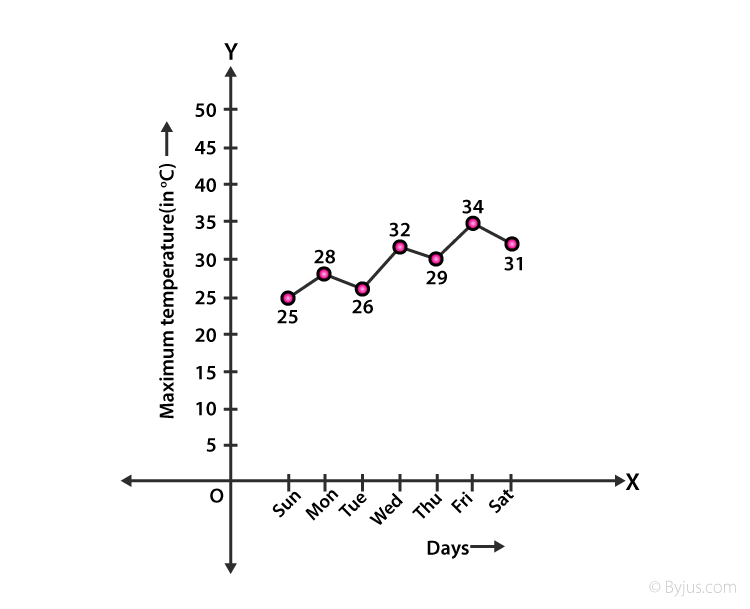2. Consider the following input/output table. Draw a line graph for it.

 Input 1 2 4 5 7 Output 2 5 11 14 20

Now, use the graph drawn to predict the outputs for the inputs of 3 and 8

Solution: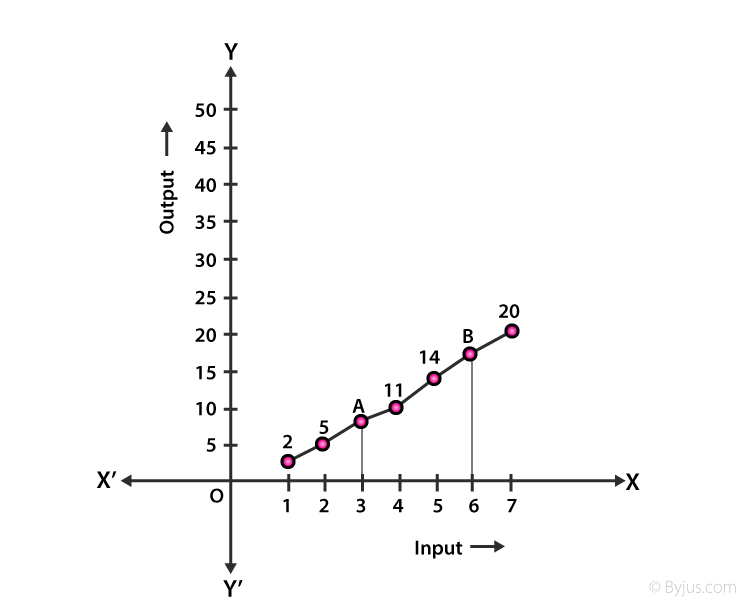From the above graph the outputs for the inputs 3 and 8 are 8 and 23.

3. The table given below depicts the annual gross profit of a company for a period of 5years.Study the table and draw a line graph for the same.

 Year 2013 2014 2015 2016 2017 Gross profit (in lakhs of ₹) 17 15.5 11.4 12.1 14.9

Solution: The line graph is represented below: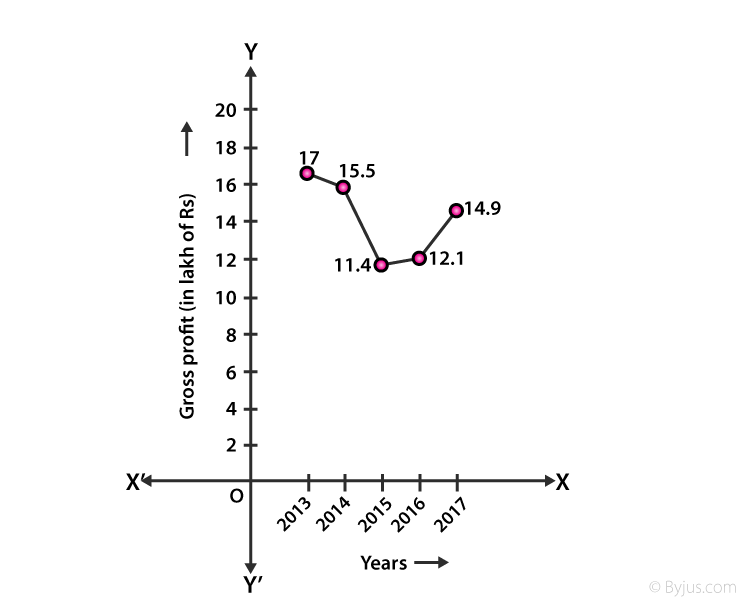4. Ajeeta starts off from home at 7 a.m. with her father on a scooter that goes at a uniform speed of 30 km/hr. Her father drops her at her school after half an hour. She stays in the school till 1.30 p.m. and takes an autorickshaw to return home. The autorickshaw has a uniform speed of 10 km/hr. Draw the line graph for the given situation and also determine the distance of Ajeeta’s school from her home.

Solution: let’s calculate the distance of ajeeta’s school from home

By using the formula,

Speed = distance/time

30 = distance/ ½

∴ The distance = 15km

We can represent it on graph as shown below.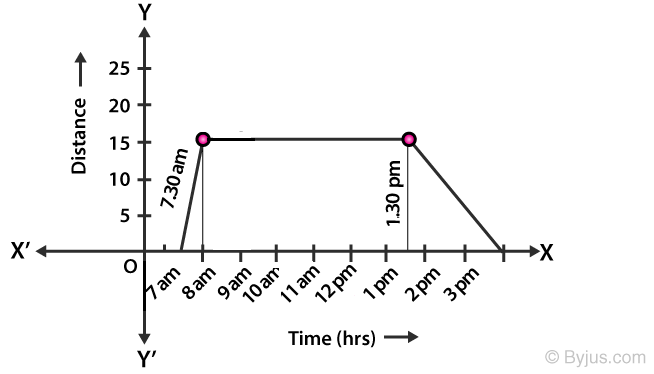5. The following table shows the percentage of students who dropped out of school after completing high school

 Year 2005 2007 2009 2011 2013 2015 2017 Percentage of students who dropped out of school 6 % 5.5% 5% 4.7% 4.9% 4% 4.5%

Study the above table carefully and draw a line graph to depict it.

Solution: The line graph is represented below: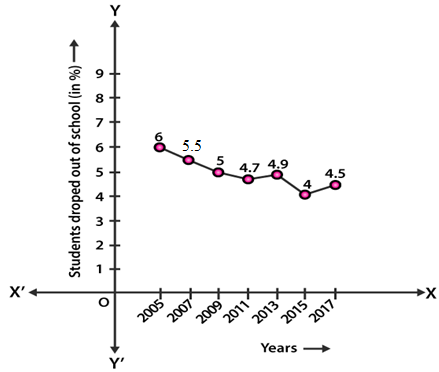6. The following chart gives the growth in height in terms of percentage of full height of boys and girls with their respective ages.

Study the table and draw the line graph of the data given below:

 Age(in years) 8 9 10 11 12 13 14 15 16 17 18 Boys 72% 75% 78% 81% 84% 88% 92% 95% 98% 99% 100% Girls 77% 81% 84% 88% 91% 95% 98% 99% 99.5% 100% 100%

Now, use the graph to answer the following questions:

(i) In which year both the boys and the girls achieve their maximum height?

(ii) Who grows faster at puberty (14years to 16years of age)?

Solution: The line graph is represented below: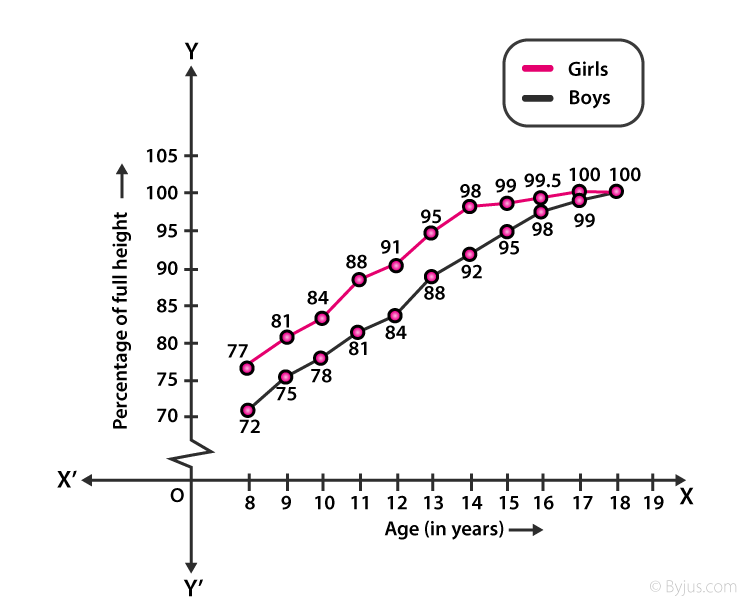(i) In the 18th year both boys and girls achieve their maximum height.

(ii) Boys grow faster than girls at puberty.

7. A car is travelling from city P to city Q, which are 350km apart. The line graph given below describes the distance of the car from the city P at different times.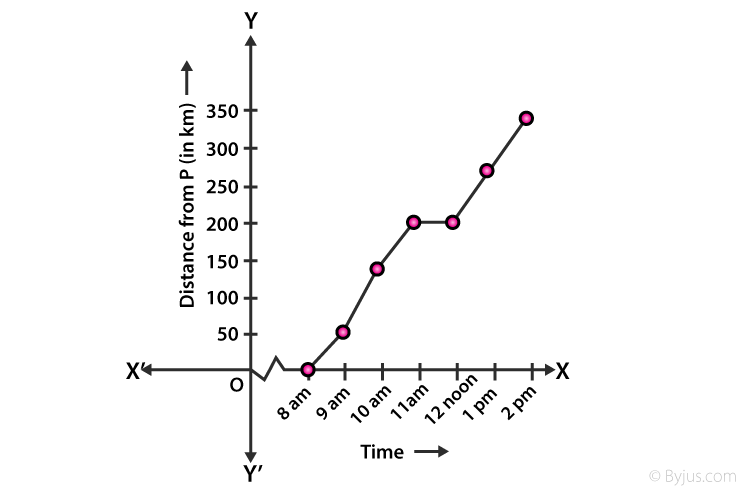Study the above graph and answer the questions given below

(i) What information is given on the two axes?

(ii) From where and when did the car begin its journey?

(iii) How far did the car go in the first hour?

(iv) How far did the car go during (a) the 2nd hour (b) the 3rd hour?

(v) Was the speed same during first three hours? How do you know it?

(vi) Did the car stop for some duration at any place? Justify your answer.

(vii) When did the car reach the city Q?

Solution:

(i) On the two axes – the x-axis shows the time and y-axis shows the distance of the car from city P.

(ii) The car begins its journey from city P at 8:00 a.m.

(iii) The car covered a distance of 50km in the 1st hour.

(iv) The car covered a distance of

(a) 100 km in the 2nd hour.

(b) 50km in the 3rd hour.

(v) No the speed was not same, because the distance covered per hour is not uniform.

(vi) Yes, the car stopped at a place during the time interval 11a.m. to 12 noon as indicated by horizontal line segment during this period.

(vii) At 2 p.m. the car reached the city Q.

8. A courier-person cycles from a town to a neighbouring suburban area to deliver a parcel to a merchant. His distances from the town at different times are shown by the given graph.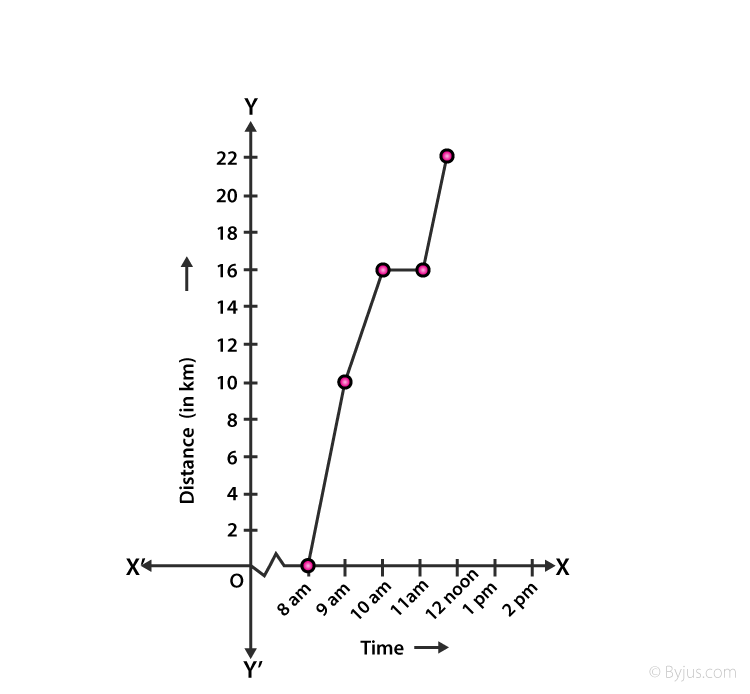Study the above graph carefully and answer the questions given below:

(i) What is the scale taken for the time-axis?

(ii) How much time did the person take for the travel?

(iii) How far is the place of the merchant from the town?

(iv) Did the person stop on his way? Explain.

(v) During which period did he ride fastest?

Solution:

(i) The scale taken for the time-axis is 1unit=15minutes.

(ii) The person took 3hours 30minutes to travel.

(iii) The distance from the town to the merchant place is 22km.

(iv) Yes, the person stopped on his way during the time interval 10a.m to 11a.m as indicated by the horizontal line in the graph.

(v) He rides fastest during the period 8a.m to 9a.m.

9. A man started his journey on his car from location A and came back. The graph given below shows his position at different times during the whole journey.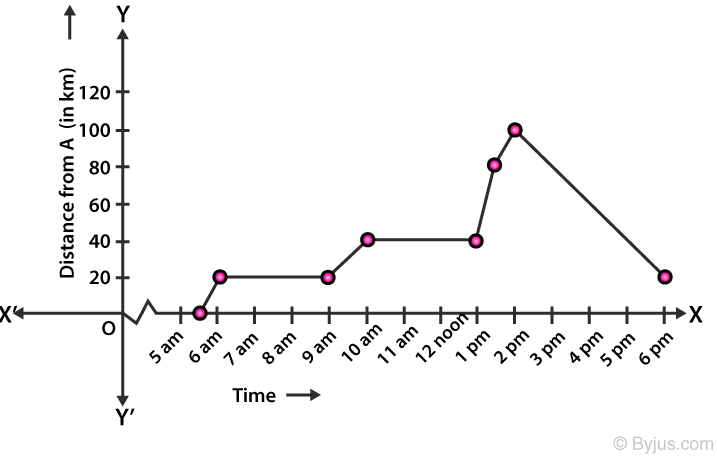Study the above graph carefully and answer the questions given below:

(i) At what time did he start and end his journey?

(ii) What was the total duration of the journey?

(iii) Which journey, onwards or return, was of longer duration?

(iv) For how many hours did he not move?

(v) At what time did he have the fastest speed?

Solution:

(i) He starts his journey at 5.30a.m and end up at 6p.m.

(ii) The total duration of the journey was 12hour 30minutes.

(iii) The onwards journey took longer duration.

(iv) He did not move for 6hours.

(v) During 2p.m to 5.30p.m period he had the fastest speed.

10. The line graph given below shows the yearly sales figures for a manufacturing company during the last five years.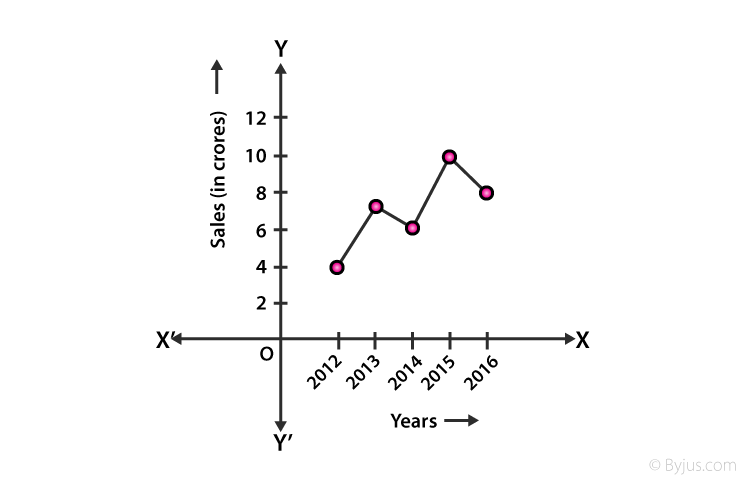Study the above graph carefully and answer the questions given below:

(i) What were the sales in (a)2013 (b)2015 (c)2016

(ii) Compute the difference between the sales in 2012 and 2016?

(iii) In which year was there the greatest difference between the sales as compared to its previous year?

Solution:

(i) The sales in (a)2013 is ₹7cr (b)2015 is ₹10cr (c)2016 is ₹8cr

(ii) The difference between the sales in 2012 and 2016 is ₹4cr

(iii) The greatest difference of sales was seen in the year 2015.

11. The following is the distance-time graph of Amit’s walking.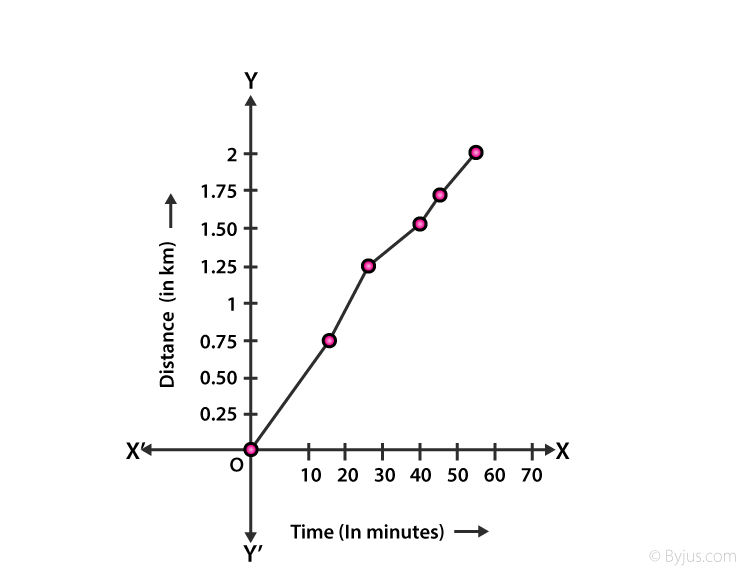Study the above graph carefully and answer the questions given below:

(ii) Find his average speed in km/hr.

Solution:

(i) As indicated by the line in the graph, Amit makes the least progress from 25 min to 40 min.

(ii) We know that the total distance covered by Amit is 2km and the time taken is 55 min i.e. 55/60hour.

By using the formula,

Average speed = total distance covered/ time taken

= 2 / (55/60) = 2/55 × 60 = 2.18 km/hour

12. For an experiment in botany, two different plants, plant A and plant B, were grown under similar laboratory conditions. Their heights were measured at the end of each week for three weeks. The results are shown by the line graph given below: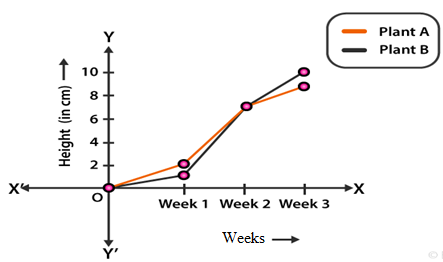Study the above graph carefully and answer the questions given below:

(i) How high was plant A after (a) 2 weeks (b) 3 weeks?

(ii) How high was plant B after (a) 2 weeks (b) 3 weeks?

(iii) How much did plant A grow during the 3rd week?

(iv) How much did plant B grow from the end of the 2nd week to the end of the 3rd week?

(v) During which week did plant A grow most?

(vi) During which week did plant B grow least?

(vii) Were the two plants of the same height during any week shown here? Specify

Solution:

(i) Plant A was (a) 7cm high after 2weeks (b) 9cm high after 3weeks.

(ii) Plant B was (a) 7cm high after 2weeks (b) 10cm high after 3weeks.

(iii) During the 3rd week plant A grew 2cm.

(iv) From the end of 2nd week to the end of 3rd week plant B grew 3cm.

(v) Plant A grew most in the 2nd week.

(vi) Plant B grew least in the 1st week.

(vii) Yes, as seen in the graph the two plants were of same height at the end of 2nd week.

## RS Aggarwal Solutions for Class 8 Maths Chapter 23 – Line Graphs and Linear Graphs

Chapter 23- Line Graphs and Linear Graphs, The RS Aggarwal Solutions present on this page provide the solutions for the questions present in the exercise. Now, let us have a look at the concepts discussed in this Chapter.

• How to draw a Line Graph?
• Double Line Graph.
• Reading the given Line Graph.
• Reading the double Line Graph.
• Linear Graph.

### Chapter Brief of RS Aggarwal Solutions for Class 8 Maths Chapter 23 – Line Graphs and Linear Graphs

RS Aggarwal Solutions for Class 8 Maths Chapter 23 – Line Graphs and Linear Graphs, ensures that the students are thorough and familiar with the concepts. Regular revision of important concepts and formulas over time to time is the best way to strengthen your concepts.

As the Chapter is about Line Graphs and Linear Graphs, here we learn how to plot the Graph, draw the line graph and read the details from the Line Graph and further solve the same. In the RS Aggarwal Solutions book, many such exercise problems are given which enhance familiarity with the concepts.